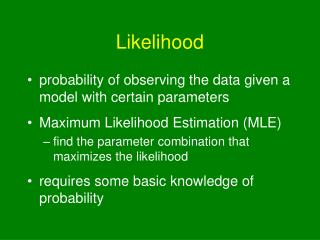DownloadDownload PresentationLikelihood

# Likelihood

Télécharger la présentation## Likelihood

- - - - - - - - - - - - - - - - - - - - - - - - - - - E N D - - - - - - - - - - - - - - - - - - - - - - - - - - -
##### Presentation Transcript

1. Likelihood • probability of observing the data given a model with certain parameters • Maximum Likelihood Estimation (MLE) • find the parameter combination that maximizes the likelihood • requires some basic knowledge of probability

2. A Poisson Example • Pr(Observation=x) = e-llx / x! • l is the parameter of the model • if Observation=4, • likelihood = e-ll4 / 4! = e-ll4 / 24

3. Multiple Observations • if two independent observations (4 & 2) • likelihood = Pr(Obs1=4) x Pr(Obs2=2) • to find the maximum • construct the likelihood equations • take the derivative(s) • solve for derivatives equal to zero

4. log-likelihood and deviance • log-likelihood (lnL) is more tractable • L = Pr1 x Pr2 x Pr3 x ... • lnL = lnPr1 + lnPr2 + lnPr3 + ... • deviance = -2*lnL